Illustrating Probability Propagation in Bayesian Networks using Local Computations

This document describes the algorithms used for probabilistic inference on a Bayesian network.  This presentation is based on the text “probabilistic Networks and Expert Systems” by R. G. Cowell, A. P. Dawid, S. L. Lauritzen, and D. J. Spiegelhalter. An example from Finn Jensen’s book “Introduction to Bayesian Networks” is used to illustrate these concepts.

. . . The premise underlying the construction of a probabilistic network is that, while the overall problem is too large … for calculating the probabilities, the individual cliques in the triangulated moral graph are of manageable size. In particular, we can perform certain operations, such as marginalization or conditioning, within any clique . . .

Let a strictly positive joint probability density for X, Y, Z  factorize as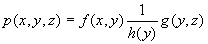(1)

The equation implies XZ|Y   and it also suggests a junction tree with two cliques and one separator: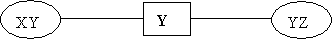We can obtain the marginal probability density p(x,y) by marginalizing over z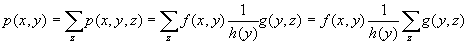(2)

Let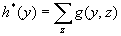(3)

Then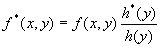(4)

The equation can be seen as “passing a message XY <- YZ via their separator,” to obtain p(x,y), where the message is expressed by h*(y), the separator is 1/h(y), and the update ratio is h*(y)/h(y).

Note that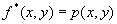,                               (5)

i.e., f*(x,y) is a marginal of p(x,y,z)

Using the marginal density f*(x,y), we can convert into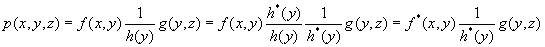(6)

Note that the new equation expresses p(x,y,z) in terms of message between the two cliques, the marginal f*(x,y), and g(y,z).

We can also obtain the marginal density for p(y,z). This would be visualized as “passing a message in the opposite direction,” i.e.,

XY -> YZ.

This is done by marginalizing over x the marginal of p(x,y,z) which we obtained in f*(x,y).  Intuitively:(7)

or opting for the hard approach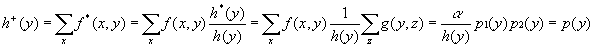(8)

Parallel to eq. 4 we calculate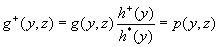(9)

i.e., we have marginalized p(x,y,z) over x. With this, we can express p(x,y,z) in terms of the new messages (compare with eq. 1):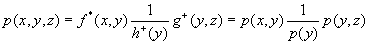(10)

“… By the passage of the two flows (messages) we have transformed the original representation of p(x,y,z) into a new one involving only marginal densities.“

Example (From Finn Jensen: An introduction to Bayesian Networks):

Holmes leaves his house. He observes that his grass is wet (H). Is it due to rain (R)? or has he forgotten to turn the Sprinkler (S) off?

Later on, as a second observation, Holmes notes that Mr. Watson grass (W) is also wet. Holmes is almost certain that it rained.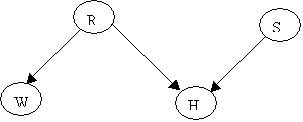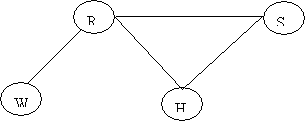The Junction Tree will be: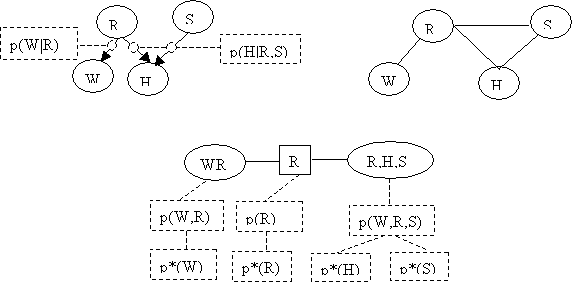Assume the following priors:

p(R) = (0.2, 0.8) [ y, n ]

p(S) = (0.1, 0.9) [ y, n ]

The conditionals are:

 R=y R=n R=y R=n W=y 1.0 0.2 S=y (1, 0) (0.9, 0.1) W=n 0.0 0.8 S=n (1, 0) (0,    1.0)

P(W|R)                        P(H|R,S)

The vectors in the table represent (H=y, H=n).

Converting P(H|R,S)  into the format we have used:

 R=y R=n S=y S=n S=y S=n H=y 1.0 1.0 0.9 0.0 H=n 0.0 0.0 0.1 1.0

From the graph we can obtain the joint probability for the CLQ(H,R,S)

P(H,R,S) = P(H|R,S)P(R,S)

Since R and S are independent (H is a collider)

P(H,R,S) = P(H|R,S)P(R)P(S)

We can obtain the prior probability table for p(H,R,S) using the expression

p(H,R,S) = p(H|R,S)p(R)p(S)

e.g.,

p(H=y,R=y,S=n)    = p(H=y|R=y,S=n)*p(R=y)*p(S=n)

= 1.0*0.2*0.9 = 0.18

p(H=n,R=n,S=n)   = 1.0*0.8*0.9 = 0.72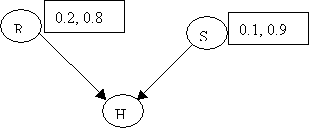Summarizing in table form:

 R=y R=n S=y S=n S=y S=n H=y 0.02 0.18 0.072 0.0 H=n 0.0 0.0 0.008 0.72

We can obtain p(H) by marginalizing R and S out of P(H,R,S)

 R=y R=n S=y S=n S=y S=n p(H) H=y 0.02 0.18 0.072 0.0 0.272 H=n 0.0 0.0 0.008 0.72 0.728

P(H) = (0.272, 0.728)

Doing the same for p(W), starting off with p(W,R)

p(W,R) = p(W|R)p(R)

p(W,R) = p(w=y|r=y)p(R=y)=1.0*0.2 = 0.20

p(W,R) = p(w=y|r=n)p(R=y)=0.2*0.8 = 0.16

p(W,R) = p(w=n|r=y)p(R=n)=0.0

p(W,R) = p(w=n|r=n)p(R=n)=0.8*0.8 = 0.64

 R=y R=n p(W) W=y 0.2 0.16 0.36 W=n 0.0 0.64 0.64

This completes the initialization phase. The network looks like:Suppose we observe that H=y. A new table p*(H,R,S) is needed.

This table is created by zeroing the entries for H=n from the table p(H,R,S), and then dividing by p(H=y)

 R=y R=n S=y S=n S=y S=n H=y 0.02*1/.272 0.18*1/.272 0.072*1/.272 0.0*1/.272 H=n 0.0*0.0 0.0*0.0 0.008*0 0.0*0

 R=y R=n S=y S=n S=y S=n H=y 0.074 0.662 0.264 0.00 H=n 0.00 0.00 0.00 0.00

We marginalize from p*(H,R,S) on (H,S) and on (H,R) to obtain

p*(R)= (0.736, 0.264) and  p*(S)=(0.339, 0.662).

Now we use the new p*(R) to update p(W,R), using

p*(W,R) = p(W|R)*p*(R) = p(W,R)[ p*(R)/p(R) ]

 R=y R=n W=y 0.2*0.736/0.2 0.16*0.264/0.8 W=n 0.0 0.64*0.264/0.8

 R=y R=n W=y 0.736 0.0528 W=n 0.0 0.2112

In summary: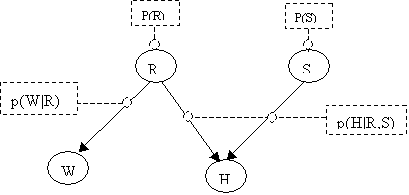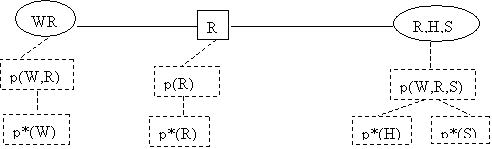Definitions

A discrete potential on U is a mapping from U. The table of conditional probabilities p(xk|Xpa(k)) forms a potential on kÈpa(k). The potential’s members are real, non-negative numbers, normalized to sum unity for each parent configuration when summed over the states of k.

[Def 1 Extension]

The authors mention two concepts on this definition:  Extension and projection.  Let UÍWÍV. Let f(.) be a potential on U. That potential is extended to W as follows: Let xÎW. Define f (x)= f (y) where y is the projection of x onto U.

[Def 2 Multiplication of Potentials]

Let f and y be two potentials. The product of the two potentials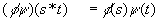is in fact just a dot product of UÈW.

 a1 a2 a3 c1 c2 c3 a1 a2 a3 B1 x1 x2 x3 d1 p1 p2 p3 b1 x1p1 x2p2 x3p3 B2 y1 y2 y3 d2 q1 q2 q3 b2 x1q1 y2q2 y3q3 B3 z1 z2 z3 d3 r1 r2 R3 b3 z1r1 z2r2 z3r3 s t s*t

Addition is defined analogously. For potentials f and y on U and W respectively, we define their sum f and y on U È W by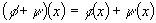Where f and y on the right hand side have been first extended to U È W.

[Def 4 Division]

Division is likewise defined in the obvious way, except with special care has to taken when dividing by zero.(11)

[Def 5 Marginalization]

Let WÍUÍV. Let f be a potential on U. The expression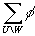is denoted as the margin, or sum-margin of f on W, and it is defined, for x Í W(12)

Where z.x is the element in W with projections x to U and z to W\U

Section 3 Local Computations on the Junction Tree

This section describes how to initialize the tree, compute clique potentials, propagate computations between adjacent cliques and beyond, and determine when equilibrium is reached.

The section also describes algorithms for two-phase propagation. All these topics are illustrated with one example (Child network) at the end of the section.

The graphical representation is generated from judgments about causal relationships that somehow are expressed as a chain graph K.

From K a junction tree T of sets of cliques is generated as described before. The sets of cliques is denoted C.

The junction tree must be initialized before it can be used. This provides a localized representation for the overall distribution.

After initialization, the tree can receive evidence, which consists of asserting some variables to specific states.  This type of evidence is known as “hard evidence.”

Local computations are performed following an orderly sequence that propagates through messages to all the cliques in the tree.

The density p(.) of the probability distribution P in the model with a chain graph K and a set of chain components K factorizes as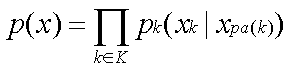(13)

and more fully as(14)

A factorization over T of the density of the joint distribution P on T is constructed.  Each clique in C and each separator in S have potentials denoted as fc  and fs . These potentials are initialized to unity, i.e. each state equals 1.0. For example the potential Y(R,H,S) in the Holmes problem is

 R=y R=n S=y S=n S=y S=n H=y 1.0 1.0 1.0 1.0 H=n 1.0 1.0 1.0 1.0

Then each factor in eq. 14 is multiplied into the potential of any clique that contains all the nodes labeling its arguments.

For example the potential Y(R,H,S) in the Holmes problem becomes, from the information in the graph, which tells us that

p(H,R,S) = p(H|R,S)p(R)p(S)

 R=y R=n S=y S=n S=y S=n H=y 0.02 0.18 0.072 0.0 H=n 0.0 0.0 0.008 0.72

After each factor has been multiplied we will have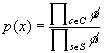(15)

where each separator potential still equals 1.

This representation is called the initial representation of P, and its potentials are called the initial potentials.

In order to understand the flow between adjacent cliques we need to define the following:

[Def 6 Charge]

A collection of non-negative potential functions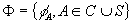is termed as a charge.

[Def 7 Contraction]

For any charge that is defined as a set of non-negative potential functions, the right-hand side of the Eq. 15 defines its contraction.

[Def 8 Generalized Potential]

Whenever Eq. 15 holds we callas a generalized potential representation of the joint distribution P on a junction tree T.

The algorithm is best described as a series of messages between adjacent cliques and their separators. Each message acts on a clique and its separator.

Let C1 and C2 be two adjacent cliques in T, and let S0 be their separator. A message (or flow) called sum-flow is described, between a source clique and a sink clique.

Assume a charge F exists,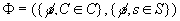.  The effect of the message will be to replace this charge by a new one, denoted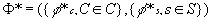.

The new potentials for the sink and the separator are given by eqs. 16, 17. Note that only the sink C2 and the separator S0 are affected by the message.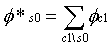(16)

where lso is called the update ratio, given by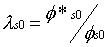(17)

A charge is unaffected by the message if the charge is sum-consistent, a condition which is defined as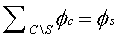(18)

Active Flows

Proposition 1: Co is a leaf. To is the subtree that resulted from separating Co from T1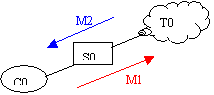By induction, for a larger tree (no separators shown, nodes are cliques)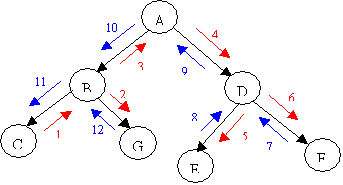Reaching Equilibrium

See the preliminary definitions in the section. A subtree T’ is live at a stage of a schedule if it has already received active messages from all its neighbors.

Theorem 1: Suppose we start with a representation F0 for a function f that factorizes T and progressively modify it by following a schedule of messages.  Then whenever T’ is live, its potential is the sum-margin fu’ of f on U’.

Corollary 1: Whenever a clique C is live, its potential is fc.

Corollary 2: Any time after active messages pass in both directions across an edge of T. the potential for its separator S is fs.

Corollary 3: Any time after active messages pass in both directions across an edge of T between cliques C1, C2, with separator S, the tree becomes sum-consistent with S, a condition defined as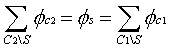(19)

Corollary 4: After passage of a full schedule of messages, the resulting charge is the marginal charge Ff of f, and the tree will be sum-consistent, which means that it has reached equilibrium.

Corollary 5: Suppose that some function f factorizes on T. Then  Ff (charge of f) is a representation for f, hence,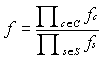(20)

Section 4 Scheduling the Messages

Inactive messages are not scheduled. There are several scheduling schemes that can be used to propagate evidence on the junction tree, all of which should produce the same results.  The main difference is the efficiency of the algorithms.

A popular scheduling scheme, due to Finn Jensen et al., is known as the two-phase propagation. An arbitrary clique is selected to become the root clique. A collection phase passes messages along the edges directed towards the root clique. The next phase, called distribution, passes messages back to the periphery starting at the root.

If A is the root: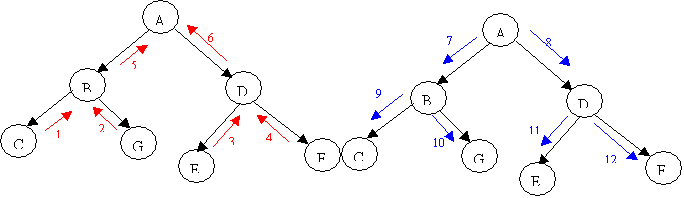Two-Phase Propagation

This scheduling scheme is due to Finn Jensen et al., from Aalborg University in Denmark.

The algorithm schedules the messages in two phases, called collection phase and distribution phase.

The specific actions taken at each phase are:

[Def 9 Collect Evidence]

This is a recursive definition. Note the distinction between calling a message and sending a message.  When CE is called in a clique U from neighbor W, U passes the call to all its neighbors except W. After all neighbors have responded to the call with a flow of their own back to U, U passes a flow back to W.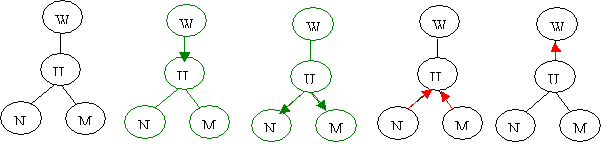At this point the root clique has absorbed all the available information from all its neighbors.  This information must be distributed in a procedure called distribute evidence, described in

[Def 10 Distribute Evidence]

When DE is called for clique U from W, a message (flow) is passed from W to U, and then U propagates the message to its neighbors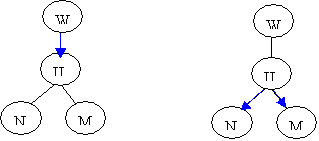Entering and Propagating Evidence

When evidence e(x) arrives to the network, it enters via the initialized junction tree. A potential containing the node associated to the new evidence is multiplied by [eq 6.19].

The new set of potentials now represents: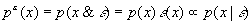The tree will not reach equilibrium until a full schedule of messages is passed amongst the cliques in both directions.

Once that is done, posterior probabilities can be found by normalizing the potentials to sum to 1, see eqs 6.20, 6.21.

A Propagation Example

This example uses a portion of a child network from the book Cowell et.al.  The conditional probabilities involved, p(n4|n2) and p(n15|n4)  are shown in Table 3.1, pp. 30 of that book. The tables are

 N4: LHV? N2 Disease? Yes No PFC 0.10 0.90 TGA 0.10 0.90 Fallot 0.10 0.90 PAIVS 0.90 0.10 TAPVD 0.05 0.95 Lung 0.10 0.90

 N15: LHV Report? N4 LHV? Yes No Yes 0.90 0.10 NO 0.05 0.95

The three nodes form a charge given by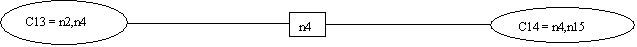The initial tables in the cliques are given by p(n4|n2) and p(n15|n4). Initially all the items in the separator are set to unity. Note that the charge is at equilibrium.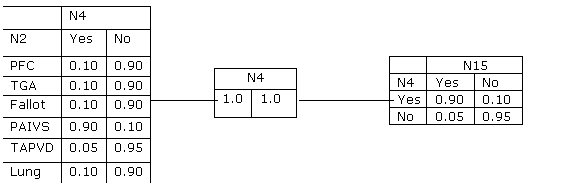1.    Evidence Yes is entered in N15, i.e., i(n15) =(1.0, 0.0).

2.    Y*(C14)=p*(n15,n4)=i(n15)´p(n15|n4)={[0.9,0.05],[0.0,0.0]}

3.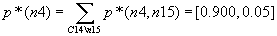4.    The update ratio is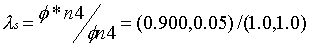5.    Y*(C13)=p*(n4,n2)=p*(n4)´ls, resulting in table at left, below: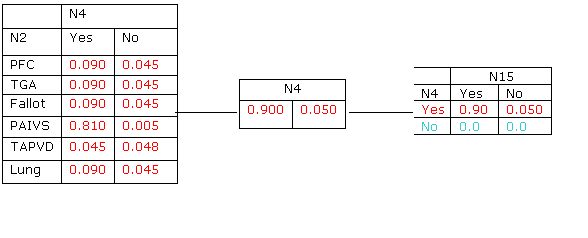6.    The propagation continues on to the parent(s) of n15 and into the rest of the junction tree. Somehow the propagation comes back into the clique C13. At this point the potential in C13 is that of table 6.1c, reproduced here, at the left:7.    The new separator is calculated by marginalizing n2 out of C13: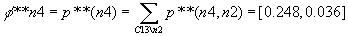8.    The new update ratio is l = ({0.248/0.9}, {0.036/0.05} )= (0.276,0.720). The new potential at C14 is propagated from n4, (0.248, 0.036).

9.    Summing all the items of any potential gives us the normalization constant a= 0.284.  Dividing each of the elements in all tables by a gives the entries in the table which is the final answer.

  The equality is not possible unless the statement of conditional independence is true.